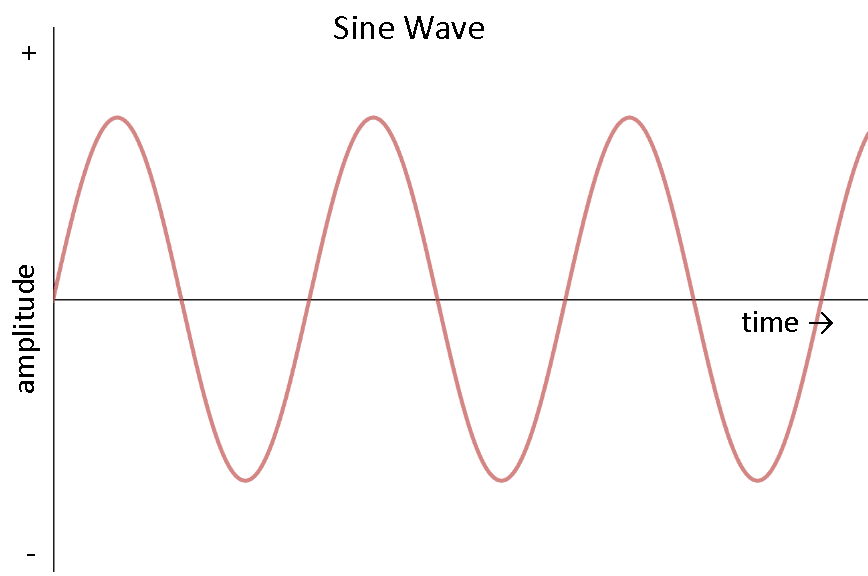# Purely Capacitive AC Circuit -- Seeking intuition for why why voltage lags behind current

Kaushik
Consider a circuit with a witch, capacitor and an AC voltage source.
The sinusoidal AC voltage source is depicted in the following graph:We know that, ##Q = CV##
##\frac{dQ}{dt} = C \frac{dV}{dt}##
##i = C\frac{dV}{dt} \tag{1}##

So from the graph, the voltage increases rapidly around ## t = 0##.
So, as ##\frac{dV}{dt}## is high (but ##V = 0##), ##i## must also be high.

Similarly, when ##V## attains a maximum, ##\frac{dV}{dt}=0##. Hence, ##i = 0##.

So we can see that current is ahead of voltage by ##\pi/2##.

But, I can't feel it. Could someone please give an intuitive explanation to this?

Last edited by a moderator:

The 'intuitive' explanation must just be that when the current is at its greatest value, the rate of flow of charges onto one end and off of the other end of the capacitor, and thus also the rate of change of capacitor voltage, is greatest. And a little bit later when the current is zero, in a subsequent small interval ##\delta t## the charge on the capacitor will be constant to first order (i.e. no charges flowing into or out of the capacitor), and the voltage is at a maximum.

But it would be best to just say that ##V_c \propto \sin{(\omega t)}## then ##i \propto \dot{V_c} \propto \cos{(\omega t)} = \sin{(\frac{\pi}{2} + \omega t)}##.

Last edited by a moderator:
•Kaushik
Staff Emeritus
Current must flow for a period of time to make the voltage change. So voltage lags current even in the case of a DC step change. Why should it be different if we let the current vary periodically?

•Kaushik, hutchphd and etotheipi
Kaushik
capacitor voltage
You use the word capacitor voltage here. I have a doubt related to this (might be silly but please excuse me).

Consider a circuit with a battery, say ##12 V##, a capacitor and a switch. As soon as we close the switch current starts to flow and this is because of the ##12V## potential difference created by the battery. But at the same instant when we close the switch, the charge stored on the plates of the capacitor is ##0##. So the electric field b/w the plates ##= 0## which implies ##V = 0##.

So, does that mean that the capacitor voltage and battery voltage should be the same at every instant?

Thanks :)

Homework Helper
Gold Member
As soon as we close the switch current starts to flow and this is because of the 12V potential difference created by the battery. But at the same instant when we close the switch, the charge stored on the plates of the capacitor is 0. So the electric field b/w the plates =0 which implies V=0.
This is a contradiction if you consider every component to be ideal. This is similar to saying "current is infinite when resistance is 0, so ohm's law fails".
Practically, there is some non-zero resistance in the wire (and in the voltage source, and in the capacitor plates, and in the switch).
There is some capacitance and inductance due to the circuit geometry and materials used. There is also a finite time delay in signal propagation in the circuit, as the signal travels at a speed close to the speed of light.

Based on your application (or problem at hand), you consider some of these effects in your circuit and ignore others.

Here you need to consider the resistance (and maybe inductance, depending on how the wires are oriented w.r.t each other) of the wires and other components. In that case, you will observe a sudden spike of current. The capacitor voltage will be equal to the battery voltage when this spike dies out.

•Kaushik and etotheipi
Gold Member
2022 Award
Intuitively you get the idea when thinking about switching on the circuit with the capacitor initially uncharged. In the very first moment the current flows (in the usual quasistationary approximation for compact geometries instantaneously) as if there is no capacitor at all while the voltage at the capacitor has to build up. It's also easy to write down the differential equation and solve it, but be aware that it's a bit difficult with zero resistance. So just take a series of a capacitor and a resistor.

•Kaushik and etotheipi
Gold Member
A mechanical analogy is a spring. If we consider spring extension the analogue of charge, and force analogous to voltage, then extension is proportional to force, and Q is proportional to V. If you connect the spring to a rotating crank, so its length follows a sinusoidal pattern, then the force (V) is proportional to the extension (Q). But the rate of change of length, which is analogous to current, is maximum at 90 degs displaced from the voltage.

•Kaushik
•